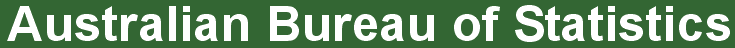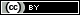ABS Home
 Statistical Language - Describing FrequenciesDescribing Frequencies

This animation explains the concept of frequencies. If you are unable to access the video a Transcript (.doc 28kb) has been provided. The animation requires Adobe Flash Player to run. The animation contains no audio.

What is a frequency?

The frequency is the number of times a particular value for a variable (data item) has been observed to occur.

How can we measure frequency?

The frequency of a value can be expressed in different ways, depending on the purpose required.

The absolute frequency describes the number of times a particular value for a variable (data item) has been observed to occur.

The simplest way to express a frequency is in absolute terms.

A relative frequency describes the number of times a particular value for a variable (data item) has been observed to occur in relation to the total number of values for that variable.

The relative frequency is calculated by dividing the absolute frequency by the total number of values for the variable.

How are relative frequencies expressed?

Ratios, rates, proportions and percentages are different ways of expressing relative frequencies.

A ratio compares the frequency of one value for a variable with another value for the variable.

The first value identified in a ratio must be to the left of the colon (:) and the second value must be to the right of the colon (1st value : 2nd value).

For example, in a total of 20 coin tosses where there are 12 heads and 8 tails, the ratio of heads to tails is 12:8. Alternatively, the ratio of tails to heads is 8:12.

A rate is a measurement of one value for a variable in relation to another measured quantity.

For example, in a total of 20 coin tosses where there are 12 heads and 8 tails, the rate is 12 heads per 20 coin tosses. Alternatively, the rate is 8 tails per 20 coin tosses.

A proportion describes the share of one value for a variable in relation to a whole.

It is calculated by dividing the number of times a particular value for a variable has been observed, by the total number of values in the population.

For example, in a total of 20 coin tosses where there are 12 heads and 8 tails, the proportion of heads is 0.6 (12 divided by 20). Alternatively, the proportion of tails is 0.4 (8 divided by 20).

A percentage expresses a value for a variable in relation to a whole population as a fraction of one hundred.

The percentage total of an entire dataset should always add up to 100, as 100% represents the total, it is equal to the ‘whole’. A percentage is calculated by dividing the number of times a particular value for a variable has been observed, by the total number of observations in the population, then multiplying this number by 100.

For example, in a total of 20 coin tosses where there are 12 heads and 8 tails, the percentage of heads is 60% (12 divided by 20, multiplied by 100). Alternatively, the percentage of tails is 40% (8 divided by 20, multiplied by 100).

 Privacy | Disclaimer | Feedback || © Copyright| Sitemap| Online Security